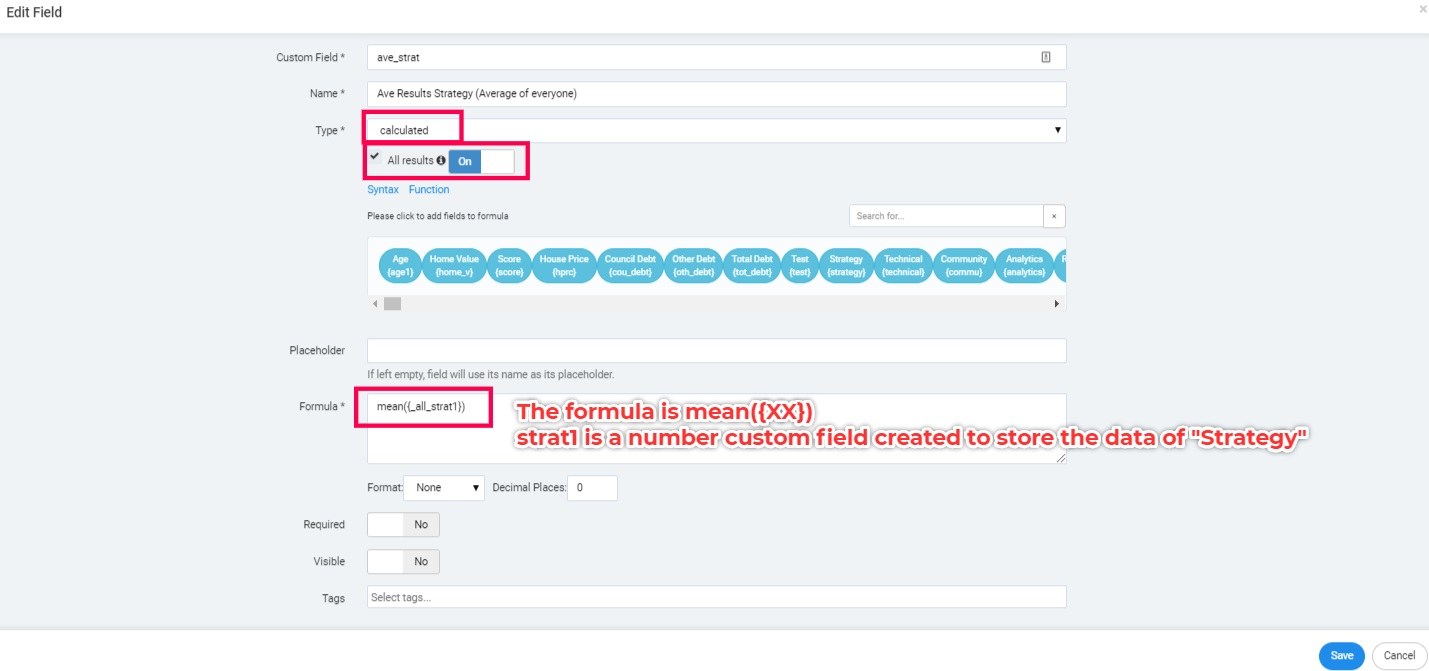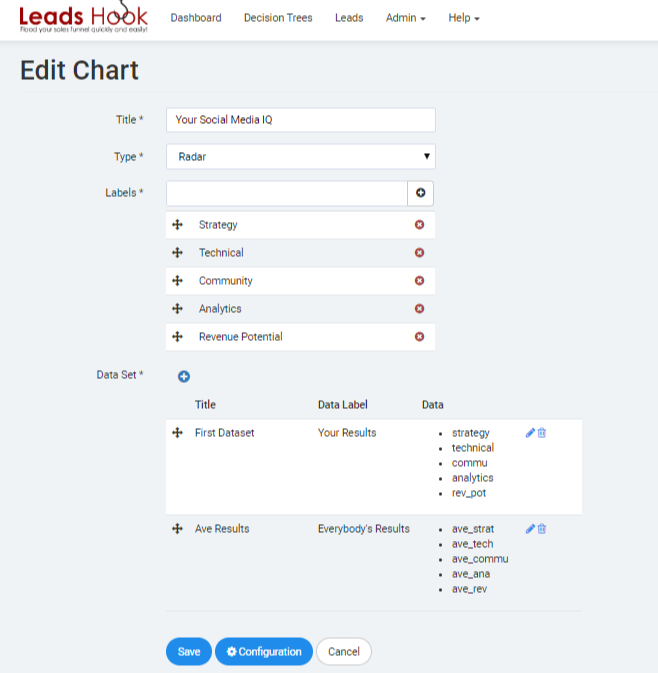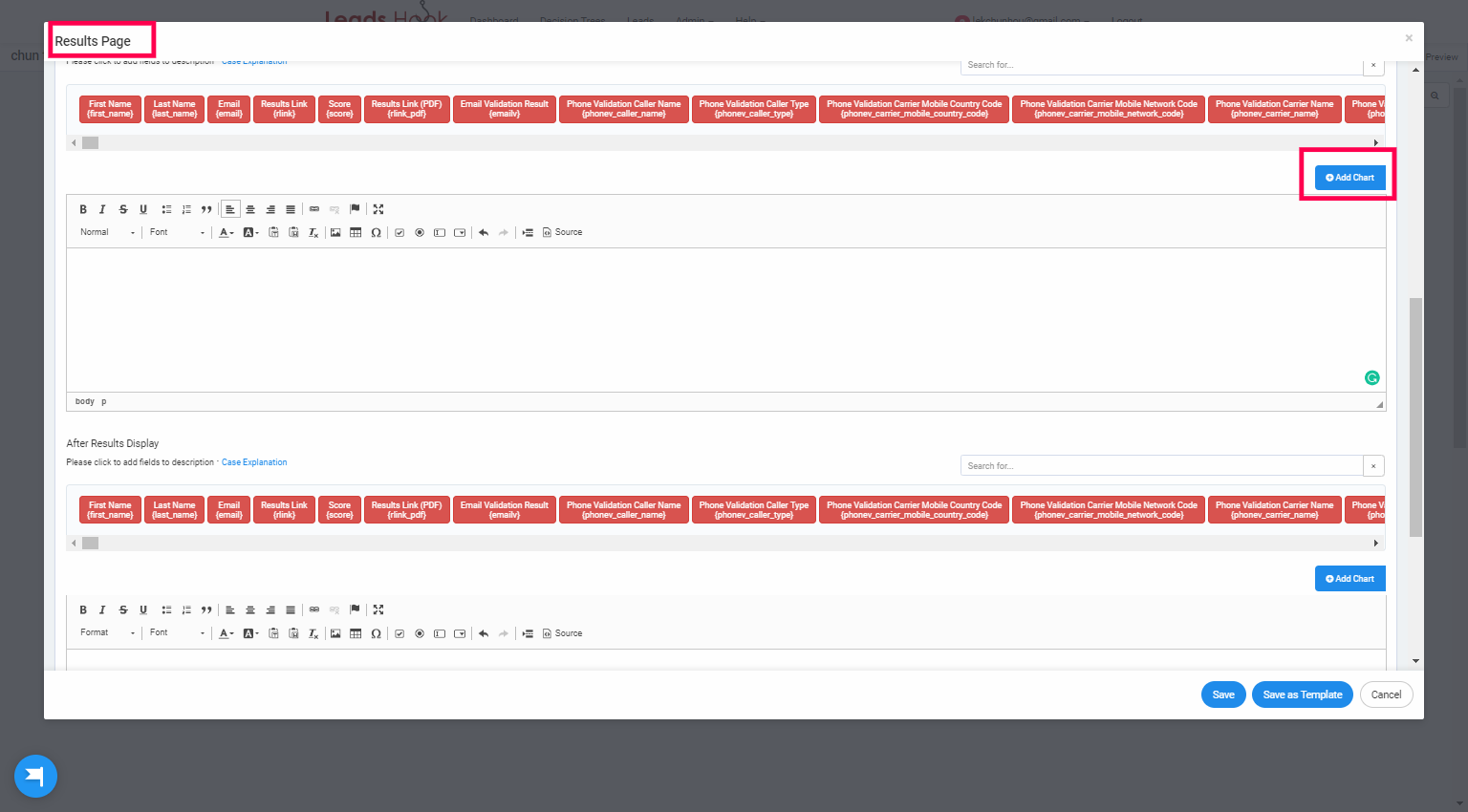# Introduction

We can compile all the results from your leads and present them in a single chart.# Step by Step Instructions

1. Create calculated custom fields to calculate the average of each data.

Based on the above examples, we will create 5 calculated custom fields to calculate the average of each data:

• Strategy
• Technical
• Community
• Analytics
• Revenue Potential

Note: Strategy, Technical, Community, Analytics and Revenue Potential data are stored in number custom fields.

Here is the configuration of a calculated custom field for “Strategy”

#